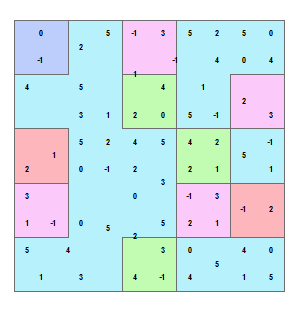# Aggregation of raster data

1922
0
09-01-2016 07:08 AM
Labels (1)byMVP Esteemed Contributor
3 0 1,922

### The aggravation of aggregation ...

Raster with some classes                                                 Aggregation using maximumNo Spatial Analyst??? A raster is just a big array... hmmmm. I wonder.

What is available at all license levels?

Not on the list?  There are examples of how to get other geometries to array format elsewhere. But everyone knows about old school ascii.  Splurge on disk space... ascii files are easy to edit.  There are many options available to get data into array format.

Code section
ASCII example...

Demo code...

This simple sample code should give you some ideas.  In this version, edge considerations are not accounted for, so should that be an issue, you can pad the array with an appropriate collar.

``"""Script:  aggregate_demo.pyModified: 2016-01-30Author:  Dan.Patterson AT  carleton.caPurpose:  To demonstrate aggregation of raster data without the spatial analyst extension.  A sample raster is created and methodsto convert an array to  a raster and vice versa are shown.Notes:- RasterToNumPyArray(in_raster, {lower_left_corner},                        {ncols}, {nrows}, {nodata_to_value})  arr = arcpy.RasterToNumPyArray(rast) - NumPyArrayToRaster(in_array, {lower_left_corner}, {x_cell_size},                    {y_cell_size}, {value_to_nodata})  rast = arcpy.NumPyArrayToRaster(a, arcpy.Point(300000,5025000),                                  10,10,-9999)  rast.save(r"F:\Demos\raster_ops\test_agg")     # esri grid, or add tif, jpg etc"""import numpy as npfrom numpy.lib.stride_tricks import as_stridednp.set_printoptions(edgeitems=3,linewidth=80,precision=2,                    suppress=True,threshold=50)from textwrap import dedentimport arcpyarcpy.env.overwriteOutput = Truedef block_a(a, block=(3,3)):    """Provide a 2D block view of a 2D array. No error checking made.    Columns and rows outside of the block are truncated.    """    a = np.ascontiguousarray(a)    r, c = block    shape = (a.shape/r, a.shape/c) + block    strides = (r*a.strides, c*a.strides) + a.strides    b_a = as_strided(a, shape=shape, strides=strides)    return b_adef agg_demo(n):    """Run the demo with a preset array shape and content.       See the header.    """    a = np.random.random_integers(0,high=5,size=n*n).reshape((n,n))    rast = arcpy.NumPyArrayToRaster(a,x_cell_size=10)    agg_rast = arcpy.sa.Aggregate(rast,2,"MAXIMUM")    agg_arr = arcpy.RasterToNumPyArray(agg_rast)    # --- a_s is the strided array, a_agg_max is the strided array max    a_s  = block_a(a, block=(2,2))    a_agg_max = a_s.max(axis=(2,3))    # ---    frmt = "\nInput array..\n{}\n\n" \          "Arcpy.sa aggregate..\n{}\n\n" \          "Numpy aggregate..\n{}\n\n" \          "All close? {}"    yup = np.allclose(agg_arr,a_agg_max)    print(dedent(frmt).format(a, agg_arr, a_agg_max, yup))    return a, agg_arr, a_s, a_agg_maxif __name__=="__main__":    """ Returns the input array, it's aggregation raster from    arcpy.sa, the raster representation of the raster and the     block representation and the aggregation array.    """    n=10    a, agg_arr, a_s, a_agg_max = agg_demo(n)‍‍‍‍‍‍‍‍‍‍‍‍‍‍‍‍‍‍‍‍‍‍‍‍‍‍‍‍‍‍‍‍‍‍‍‍‍‍‍‍‍‍‍‍‍‍‍‍‍‍‍‍‍‍‍‍‍‍‍‍‍‍‍``

This is a sample ascii file so that you can

see the numeric inputs and structure of

the ascii header and its data.

``ncols         10nrows         10xllcorner     300000yllcorner     5025000cellsize      10NODATA_value  -99990 0 2 5 -1 3 5 2 5 0 -1 -1 2 1 1 -1 -1 4 0 4 4 5 5 5 1 4 1 1 2 2 1 1 3 1 2 0 5 -1 2 3 1 1 5 2 4 5 4 2 5 -1 2 1 0 -1 2 3 2 1 5 1 3 1 5 5 0 3 -1 3 -1 2 1 -1 0 5 2 5 2 1 -1 2 5 4 4 5 2 3 0 5 4 0 1 1 3 5 4 -1 4 5 1 5‍‍‍‍‍‍‍‍‍‍‍‍‍‍‍‍``

Does it work with big rasters?

Does it support other summary statistics?

Of course, I covered the details of raster statistics in an earlier post.

That's all for now...